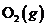Chapter 10.6, Problem 10.5SC### Introductory Chemistry: A Foundati...

9th Edition
Steven S. Zumdahl + 1 other
ISBN: 9781337399425

#### Solutions

Chapter
Section### Introductory Chemistry: A Foundati...

9th Edition
Steven S. Zumdahl + 1 other
ISBN: 9781337399425
Textbook Problem
5 views

# ercise 10.5 The reaction that occurs in the heat packs used to treat sports injuries ismg src=Images/HTML_99425-10.6-10.5SC_image001.jpg alt="" align="top"/>w much heat is released when 100 g of Fe(s) is reacted with excessInterpretation Introduction

Interpretation:

The amount of heat which is released when 1.00 g of Fe(s) is reacted with excess O2(g) should be calculated.

Concept Introduction:

Number of moles is equal to the ratio of given mass to the molar mass.

The mathematical expression is given by:

Number of moles = given massmolar mass

Now, according to the reaction, 1652 kJ heat is released when 4 moles of Fe reacts, thus by determining the number of moles of 1.00 g of iron and then multiply the number of moles of iron by heat released per four moles of iron. Hence, one can get the heat released when one mole of iron reacts.

Explanation

Given information:

4Fe(s)+3O2(g)2Fe2O3(s)

ΔH=1652 kJ

According to the given reaction, four moles of iron reacts with three moles of oxygen and gives two moles of iron(III) oxide.

First, calculate number of moles of 1

### Still sussing out bartleby?

Check out a sample textbook solution.

See a sample solution

#### The Solution to Your Study Problems

Bartleby provides explanations to thousands of textbook problems written by our experts, many with advanced degrees!

Get Started